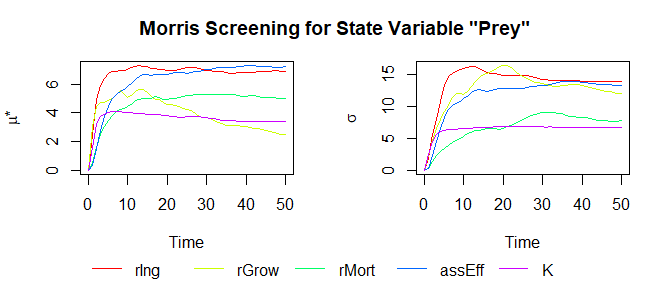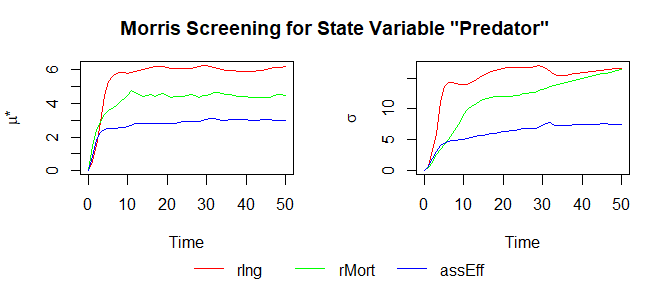# ODEsensitivity

## Introduction

The goal of sensitivity analysis is to examine how sensitive a mathematical model responds to variations in its input variables. Here we focus on the sensitivity analysis of ordinary differential equation (ODE) models via Morris screening.

If the assumption of a uniform distribution on the domain intervals doesn’t hold, the Morris screening method cannot be used and the variance-based Sobol’ method should be considered instead. In this case, simply switch from using the function ODEmorris to the function ODEsobol.

## Analyse the Lotka-Volterra Equations

The Lotka-Volterra equations describe a predator and its prey’s population development and go back to Lotka (1925) and Volterra (1926). The prey’s population at time $$t$$ (in days) will be denoted with $$P(t)$$ and the predator’s (or rather consumer’s) population with $$C(t)$$. $$P(t)$$ and $$C(t)$$ are called state variables. This ODE model is two-dimensional, but it should be noted that ODE models of arbitrary dimensions (including one-dimensional ODE models) can be analyzed with ODEsensitivity.

### Model Definition

Now we define the model according to the definition in deSolve::ode().

LVmod = function(Time, State, Pars) {
with(as.list(c(State, Pars)), {
Ingestion <- rIng * Prey * Predator
GrowthPrey <- rGrow * Prey * (1 - Prey/K)
MortPredator <- rMort * Predator
dPrey <- GrowthPrey - Ingestion
dPredator <- Ingestion * assEff - MortPredator
return(list(c(dPrey, dPredator)))
})
}

Each of the five parameter names, their lower and upper boundaries, the initial values for the state variables and the timepoints of interest are saved in separate vectors:

LVpars = c("rIng", "rGrow", "rMort", "assEff", "K")
LVbinf = c(0.05, 0.05, 0.05, 0.05, 1)
LVbsup = c(1.00, 3.00, 0.95, 0.95, 20)
LVinit = c(Prey = 1, Predator = 2)
LVtimes = c(0.01, seq(1, 50, by = 1))

### Sensitivity Analysis

The sensitivity analysis of a general ODE model can be performed by using the generic function ODEsensitivity::ODEmorris().

set.seed(1618)
LVres_morris = ODEmorris(mod = LVmod, pars = LVpars, state_init = LVinit
, times = LVtimes, binf = LVbinf, bsup = LVbsup
)

Let’s take a look at the output LVres_morris.

str(LVres_morris, give.attr = FALSE)
#> List of 2
#>  $Prey : num [1:16, 1:51] 1.00e-02 -1.90e-02 2.42e-02 4.78e-05 -3.24e-05 ... #>$ Predator: num [1:16, 1:51] 0.01 0.009441 0.000063 -0.01796 0.009611 ...

The first row of each state variable contains a copy of all timepoints. The other rows contain the Morris sensitivity indices $$\mu$$, $$\mu^\star$$, and $$\sigma$$ for all 5 parameters and all 51 timepoints.

### Plotting

ODEsensitivity provides a plot() method for objects of class ODEmorris:

plot(LVres_morris)plot.ODEmorris() has two important arguments: pars_plot and state_plot. Using pars_plot, a subset of the parameters included in the sensitivity analysis can be selected for plotting (the default is to use all parameters). state_plot gives the name of the state variable for which the sensitivity indices shall be plotted (the default being the first state variable):

plot(LVres_morris, pars_plot = c("rIng", "rMort", "assEff"), state_plot = "Predator")